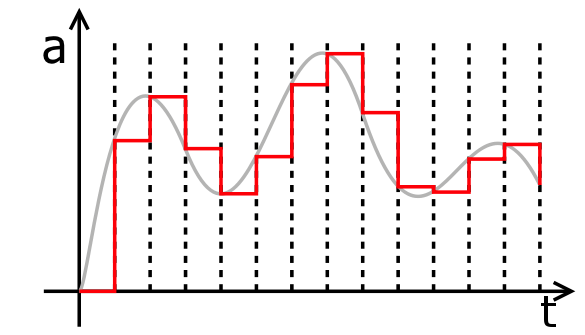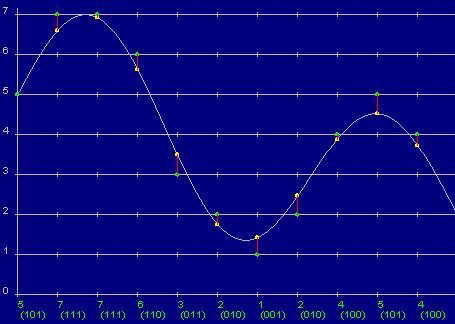# Difference between Sampling and Quantization

Sampling and quantization are two methods discussed in digital signal processing, particularly during the digitalizing of an analog signal. Both these methods are basically used in the discretization of the analog signal, which is to be converted into a digital signal so that it can be handled by computers or other digital systems. Though both of these processes sound similar, there are some distinguishing features that make them different from each other.

The basic difference between these two processes is which axis or variable is discrete and which is continuous. In sampling, the values on y-axis, usually amplitude, are continuous but the time or x-axis is discretized, whereas, in quantization, time is continuous and the y-axis or amplitude is discretized.

In sampling, one value of amplitude is selected from different values of time to represent it, while in the case of quantization, different values of time are rounded off to get a defined set of possible values of amplitude. Furthermore, sampling is always done before carrying out the quantization process.

Sampling reduces a continuous curve (Time-Amplitude graph) to a series of “tent poles” over time. Quantization, on the other hand, reduces a continuous curve to a continuous series of “stair steps” that exist at regular time intervals.

### Instructions

• 1

Sampling

“In digital signal processing, sampling is the process of breaking up a continuous signal to a discrete signal”. This method is commonly used in the conversion of a sound signal from analog to digital. The process breaks up the analog sound waves, with continuous values of amplitude, into intervals along the time axis to produce a sequence of signals. As a result, the values on x-axis (time intervals) are converted from continuous, to discrete values, with each Time interval corresponding to a specific value of amplitude.

So, instead of having a continuous analog signal, a new digitalized signal is produced with single maximum amplitude, representing the whole time interval. The resulting signal is called the Pulse Amplitude Modulated Signal, which is half continuous and cannot be represented digitally. So, a second step of discretization, called quantization, is carried out.

Image courtesy: music.cornell.edu• 2

Quantization

“In digital signal processing, quantization is the process of mapping a larger set of values to a smaller set”. This process is usually carried out to digitally represent a partially discretized signal with a single continuous value of amplitude representing each interval of time.

In this process, the continuous amplitude values are either rounded up or down to the nearest predetermined values, to get a finite set of values rather than an infinite number of values. This type of signal is called the Pulse Code Modulated Signal.

Image courtesy: telecomabc.com•